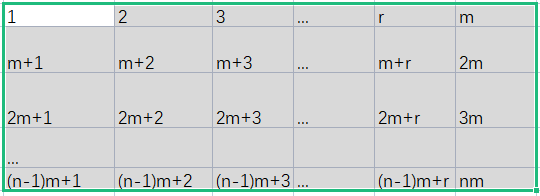# 一、RSA算法

RSA是非对称加密算法中的代表，它的重要性不言而喻，为了弄清楚RSA算法，我们一起来完成一项任务：

1、先搞清楚RSA的算法步骤

2、对每一个数字加密

3、对方能够解密

RSA的算法步骤：

1、随机选两个不相等的质数pq

2、计算pq的乘积n

3、计算n欧拉函数 φ(n)

4、随机选择一个整数e，条件是1<e<φ(n),e与φ(n)互质

5、计算e对于φ(n)模反元素d

6ne为公钥，nd为私钥

1、选两个质数 137

2、n=13*7=91

3、φ(143)=φ(13*7)=φ(13)*φ(7)=12*6=72

4、随机取一个数为5

5、通过扩展欧几里得算法，可以得出d=29

6、公钥：(91,5)   私钥：（91,29

m ^e c (mod n)

m=10532 7311111810132117

c ^d m (mod n)

c=1424776,27, 82,2,52

1、当n比较小的时候，要加密的数比n大时，可能存在解密出来的数和原来的数差一个模。比如111，加密后是76，解密后是20, 20+91=111，其中91是模

2、模反元素求出的结果是负数

负数+

## 二、质数

13岁读大学、15岁大学毕业、16岁获得硕士学位，18世纪最杰出的数学家之一，不满十岁，就开始自学《代数学》，1766年患白内障，几乎失明。

a）任何一个＞=6之偶数，都可以表示成两个奇质数之和。

(b) 任何一个＞=9之奇数，都可以表示成三个奇质数之和。

9+9开始，逐步减少每个数里所含质数因子的个数，直到最后使每个数里都是一个质数为止，这样就证明了哥德巴赫猜想。中国的数学家在这个问题上是领先世界的。

## 三、欧拉函数

Φ(m)=s，s为缩系的个数GCD(km+r,m)=GCD(r, m)，所以每一列的 n 个元素同时与 m 互素，当且仅当 GCD(r,m)=1

m互素的列共有Φ(m)个，假设第r列与m互素，r,m+r,2m+r,  (n-1)m+r mod n的一个完系，在这一列中与n互素的共有Φ(n)个，所以Φ(mm)=Φ(m)*Φ(n)

mφ(n) 1 (mod n)

它能做什么？简化幂的模运算，还能做什么，与RSA算法有什么关系？这个后面就会知道

x1,x2...xφn n互质

ax1 (mod n),ax2 (mod n)...axφn ( mod n) n互质，经过排序后与x1,x2...xφn相同

(aφ(n-1)X1 * X2 * ...* Xφn ≡ 0 (mod n)

abc为任意3个整数,m为正整数，且(m,c)=1，则当a·cb·c(mod m)时，有ab(mod m)

m是一个整数m>1b是一个整数(m,b)=1。如果a,a,a,a,…a[m]是模m的一个完全剩余系，则b·a,b·a,b·a,b·a,…b·a[m]也构成模m的一个完全剩余系。

P={1,2,3,...,p-1}

A={a,2a,3a,...,(p-1)a},也是p的一个完全剩余系。由完全剩余系的性质

a*2a*3a*...*(p-1)a1*2*3*...*(p-1) (mod p)

(p-1)! *ap-1(p-1)! (mod p)

((p-1),p)=1,两边同时约去 (p-1)!

ap-11(mod p)

## 四、模反元素

ed ≡ 1 mod φ(n)，已知e和φ(n)，求d，用扩展欧几里得算法

ed=kφ(n)+1

ed-kφ(n)=1

a*x+b*y = gcd(a , b)

= gcd(b , a mod b)

= b * x1 + (a mod b) * y1

= b * x1 + (a - a / b * b) * y1 即

a*x+b*y = b * x1 + (a - a / b * b) * y1

a*x+b*y = a*y1 - b*a/b*y1 + b*x1 ， 即

a * x + b * y

= a * y1 + b * (x1-a/b*y1)

x=y1

y=x1 - a/b*y1

void exgcd(int a, int b, int &d, int &x ,int &y)
{

if ( !b )
{

d = a;

x = 1;

y = 0;

return;
}

int x1,y1;

exgcd( b , a % b , d , x1 , y1 );

x = y1;

y = x1 - ( a / b ) * y1;

return ;
}

《孙子算经》中有这样一个问题：有物不知其数，三三数之剩二，五五数之剩三，七七数之剩二。问物几何？

x  mod 3=2

x  mod 5=3

x  mod 7=2

x1 mod 3=2

x2 mod 5=3

x3 mod 7=2

x2+x3  mod 3 = 0

x1+x3  mod 5 = 0

x1+x2  mod 7 = 0

35m1 2 (mod 3)

21m2 3(mod 5)

15m32(mod 7)

35w1 1 (mod 3)

21w2 1(mod 5)

15w31(mod 7)

## 五、解密证明

me= kn+c

c=me-kn

(me-kn)d m (mod n)

ed=hφ(n)+1

mhφ(n)+1 m (mod n)

mφ(n))h  *m m (mod n)

m,n 不互质的情况，这里不再陈述，我觉得比较繁琐。

me c (mod n)    cd m (mod n)

med m (mod n)

med-1 1 (mod n)

ed=kφ(n)+1

1、第一部分的例子中，由于n选的不够大，所以导致加密比n的数字后，解密出来后就不准了。这个问题，希望真正想学习RSA算法的益友，可以换个稍微大一点的n，再手动走一遍算法。

2、网上参考的文章都是先介绍数学知识，然后很多人可能连算法的全貌没有看到，直接退出去了，因为数学知识不容易懂。所以我反其道而行之，先体验加密解密的过程，然后再去弄懂算法背后的数学知识。

3、为了搞清楚RSA算法，我准备了好几周，补习数学知识，这些知识是算法的基石。RSA算法的可靠性，建立在大数难以分解的数学原理之上。

4、例子要传输的原文是 i love u的ASCII码，包括中间的空格

https://www.cnblogs.com/zylAK/p/9569668.html

https://zhuanlan.zhihu.com/p/58241990

https://zhuanlan.zhihu.com/p/48249182

https://zhuanlan.zhihu.com/p/103394468

posted @ 2021-08-10 18:09  micDavid  阅读(225)  评论(0编辑  收藏  举报